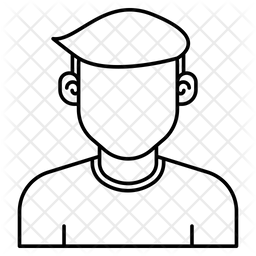# Effect size in RM ANOVA: f or d?

560 viewsHi,

I have been trying to determine the sample size for a repeated measures design with one repeated measures variable with two levels.

First, I tried with the t-test (https://webpower.psychstat.org/models/means01/), with power = 0.80, alpha = 0.05, two sided, type = paired, and a medium effect size of d = 0.50 (I assumed that the effect size was Cohen's d because of the outcome of the analysis and the man page of the R package). The result is n = 33.37, which is consistent with pwr package in R.

I also tried to replicate the analysis above with a repeated measures ANOVA (https://webpower.psychstat.org/models/means05/). My understanding is that for a within participants design with one variable with two levels, if parameters are the same the results (i.e., the expected n) should be the same as with the t-test.

For one group and two measures (that is, only one RM factor with two levels), power = 0.80, type of effect = within, and leaving sample size empty, for a medium effect of Cohen's f = 0.25 (equivalent to a d = 0.50) the outcome is that I would need 127.5 participants. Interestingly, if I enter f = 0.50 the outcome is n = 33.37, as with the t-test.

It also happens the other way round: in the t-test power analysis, if we introduce d = 0.25 the resulting n is 127.5. Thus, it seems that Cohen's d in the t-test and Cohen's f in the RM ANOVA are the same, when actually they are different (f = d / 2).

I wonder if I misunderstood the effect size of the RM ANOVA and, instead of f, it is a d, or there is any other difference between the t-test and the RM ANOVA in this particular case. Could you, please, confirm whether in the RM ANOVA we should enter Cohen's f or Cohen's d as effect size? If it's Cohen's f, why the discrepant results between the t-test and the RM ANOVA?by johnny (3.3k points)